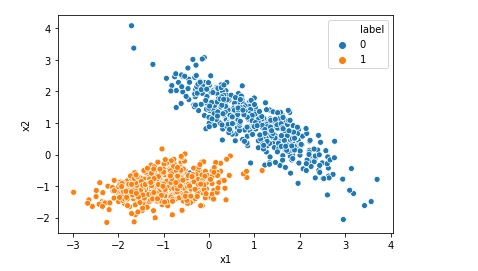# Scaling Data Range using Min Max Scaler

Suppose you have a dataset that has float values and all values in the range 0 to 1. You want to change all values to integer with a range between 10 to 20. In this post we will learn how to do this using MinMaxScaler Data before scaling Now let us scale the data as … Continue reading Scaling Data Range using Min Max Scaler# Creating Synthetic Data for Logistic Regression

Many a times we want to implement Logistic Regression on certain data but we do not find that kind of data online. In that case we can generate a synthetic data for our problem. In this post we will see how to generate a typical synthetic data for a simple Logistic Regression. Import the required … Continue reading Creating Synthetic Data for Logistic Regression# Creating Synthetic Data for Linear Regression

Many a times you want to implement Linear Regression on certain data but you do not find that kind of data online. In that case you can generate a synthetic data for your problem. In this post we will see how to generate a typical synthetic data for a simple Linear Regression. Import the required … Continue reading Creating Synthetic Data for Linear Regression# Scatter Plot using Matplotlib in Python

A scatter plot is a type of plot or graph that uses two coordinates axis (say x and y) to display values for two variables for a set of data. We can use matplotlib library of python to create scatter plot in Jupyter notebook. Let us import the required libraries first: #Import the libraries import … Continue reading Scatter Plot using Matplotlib in Python